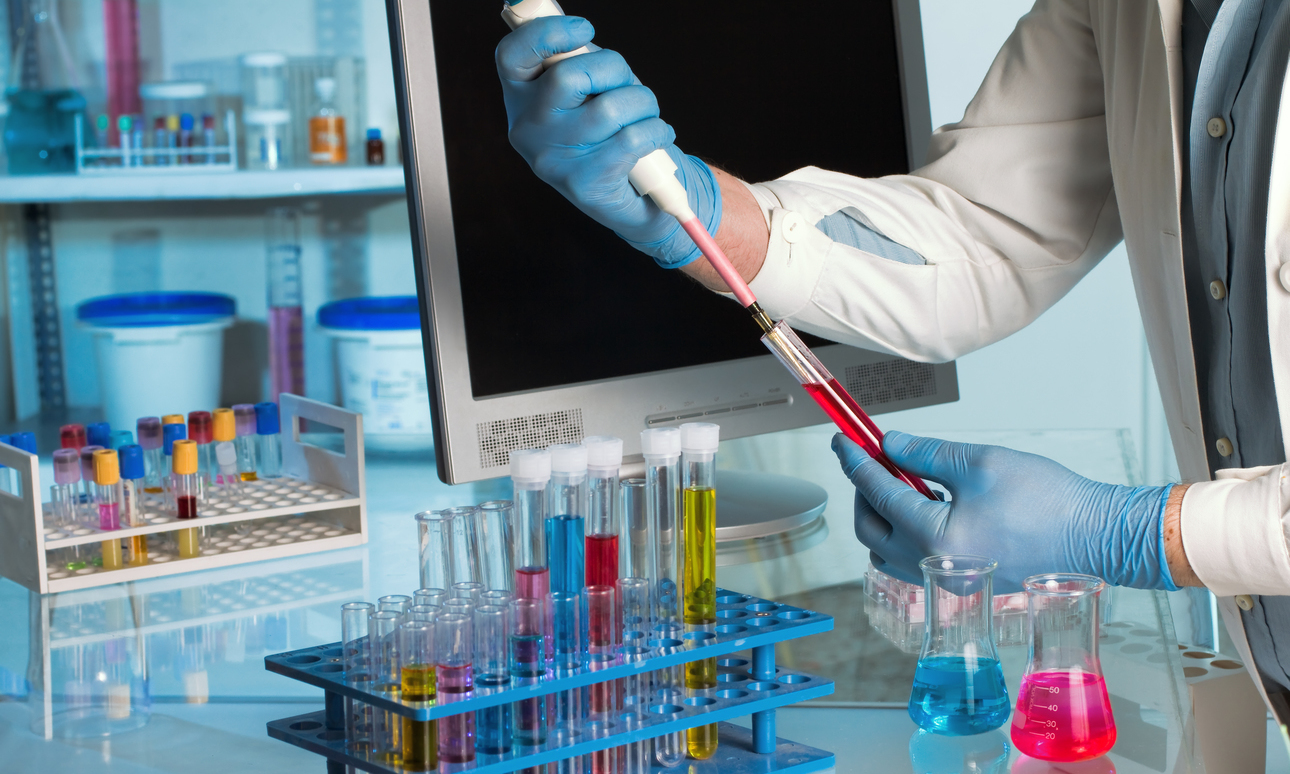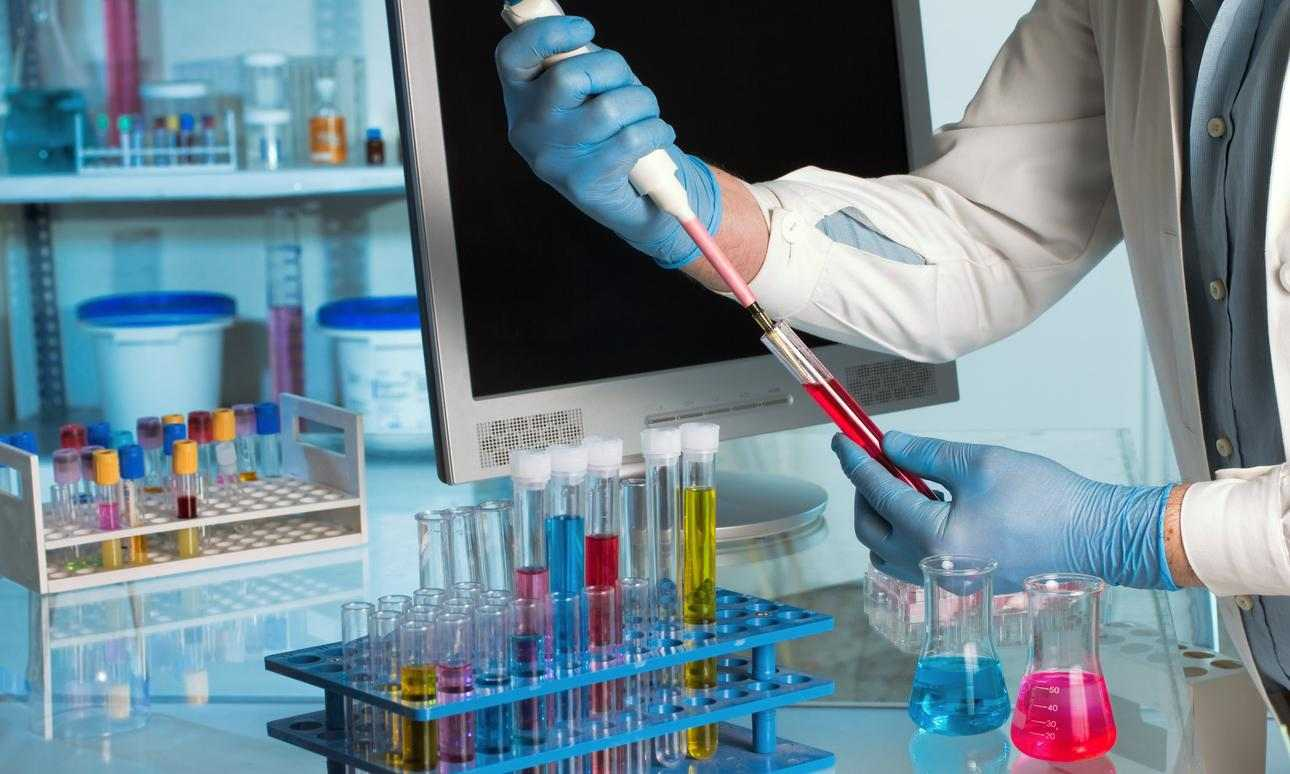# How to Correctly Monitor Reused Caustic ConcentrationRecycling and reuse of caustic (Sodium Hydroxide) is commonplace in the food, beverage and dairy processing industries as it reduces wastewater load, is more sustainable and environmentally friendly, and reduces costs.

Fresh Sodium Hydroxide is added to the recovered solution until the required cleaning chemistry strength is achieved. The typical method to determine the strength is to take a titration of a sample of the used solution with an acid of known concentration e.g. Sulfuric or Hydrochloric Acid, using Phenolphthalein as an indicator. The strength of Sodium Hydroxide is expressed as per cent Sodium Hydroxide (% NaOH) or grams of Sodium Hydroxide in 100ml.

### 2NaOH + CO2 ---> Na2CO3 + H2O

Unfortunately, the simple titration with Phenolphthalein does not distinguish between Sodium Carbonate and Sodium Hydroxide and could return false test results, impacting the efficacy of the cleaning solution.

In situations where the presence of carbonates is suspected, it is very important to distinguish how much of the solution is really Sodium Hydroxide (free caustic) and how much is actually Sodium Carbonate. A simple modification to the Phenolphthalein titration can be made by introducing a second indicator: Bromocresol Green.

### Testing Method for Recovered Caustic

The method below, taken from the Diversey Application Methods Database, describes exactly how to use these indicators and the titrating acid.

Diversey Sector Specialists can perform the procedure below, or train onsite personnel, to distinguish between the concentrations of Sodium Hydroxide (caustic) and Sodium Carbonate in the use solution.

1. Obtain a 10ml sample of the solution.
2. Add 3 drops of Phenolphthalein Indicator and mix. The solution should be pink.
3. Add 2.56N Sulfuric Acid, drop by drop, mixing after each addition and counting the number of drops until the color changes from pink to colorless.
4. Record the volume when the solution turns from pink to colorless (A).
5. Add 3 drops of Bromocresol green and mix. The solution will now turn blue if carbonates are present.
6. Starting counting from zero, add the 2.56N Sulfuric Acid drop by drop with mixing until the solution color changes from blue to yellow.
7. Record the volume (B)

### Calculation:

Free Caustic % (as NaOH)w/v = (A - B) x 1.024

Carbonate % (as Na2CO3)w/v = (B) x 2.714

Other variations of this test may be encountered in the field. One of the more common involves the use of Barium Chloride. In this method, two samples labeled A and B, each 10.0ml are taken. Barium Chloride is added to sample A. If any carbonate is present this will precipitate as Barium Carbonate leaving behind any free caustic.

Sample A is titrated with standard acid using Phenolphthalein as the indicator, giving the Hydroxide content. Sample B is titrated with standard acid using Bromophenol blue as the indicator. By subtracting the acid volume of titration A from the acid volume of B, the carbonate can be obtained.

### Measuring the Concentration of Sodium Hydroxide by using Conductivity

In the field, the concentration of Sodium Hydroxide (free caustic) can be measured indirectly by using conductivity.

The use of conductivity to record the concentration of free caustic is acceptable if the use solution is mostly Sodium Hydroxide with little Sodium Carbonate, for example, a use solution of 3% Sodium Hydroxide (NaOH) and a 1% Sodium Carbonate (Na2CO3). This is possible because at the same concentration the conductivity of Sodium Hydroxide is much larger than that of Sodium Carbonate.

However, keep in mind that the measurement of conductivity is not specific to Sodium Hydroxide. Therefore, high concentrations of Sodium Carbonate do interfere and give false readings for the concentration of Sodium Hydroxide (NaOH). For example, a solution containing 5% Sodium Carbonate and 0% Sodium Hydroxide is sensed as a 1% solution of Sodium Hydroxide.